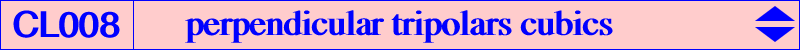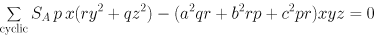Given an isoconjugation with pole W = (p : q : r), the locus of point M such that the trilinear polars of M and its isoconjugate M* are perpendicular is a nK with pole W and root R = (SA p : SB q : SC r). Its equation is :All these cubics pass through W and A, B, C, G. The tangents at A, B, C are independent of W (they join a vertex of ABC to the foot of the orthic axis on the opposite sideline). Thus, these cubics form a net. With W = G, we obtain the Simson cubic = K010 = perpendicular tripolars isotomic cubic and with W = K, we obtain the perpendicular tripolars isogonal cubic K107. The net contains only one circular cubic and only one K60, both with rather complicated poles. The cubic is a nK0 if and only if W lies on the circumcircle, and then its root R is the orthocorrespondent of its pole W. R lies on the circum-conic with center K which is the isogonal transform of the orthic axis. In this case, the cubic always contains the common points X(1113), X(1114) of the circumcircle and the Euler line. Hence, all these nK0 form a pencil of cubics. K106 is an example of such nK0.